# Monetone B-spline Smoothing

##### Posted on Mar 09, 2021 (Update: Mar 12, 2021) 0 Comments

This note is based on He, X., & Shi, P. (1998). Monotone B-Spline Smoothing. Journal of the American Statistical Association, 93(442), 643–650., and the reproduced simulations are based on the updated algorithm, Ng, P., & Maechler, M. (2007). A fast and efficient implementation of qualitatively constrained quantile smoothing splines. Statistical Modelling, 7(4), 315–328.

$\newcommand\RMSE{\mathrm{RMSE}}$

## Introduction

There are various smoothing techniques:

• kernel smoothing
• nearest neighbors
• smoothing splines
• local polynomials
• B-spline approximation
• neural networks

Focus: estimate regression curves that are known or required to be monotone.

Examples:

• growth curves, such as weight or height of growing objects over time
• item characteristic curve in the item response theory, which measures the probability of getting a correct answer for an examinee with given latent ability parameter.

### Item-response theory (IRT) model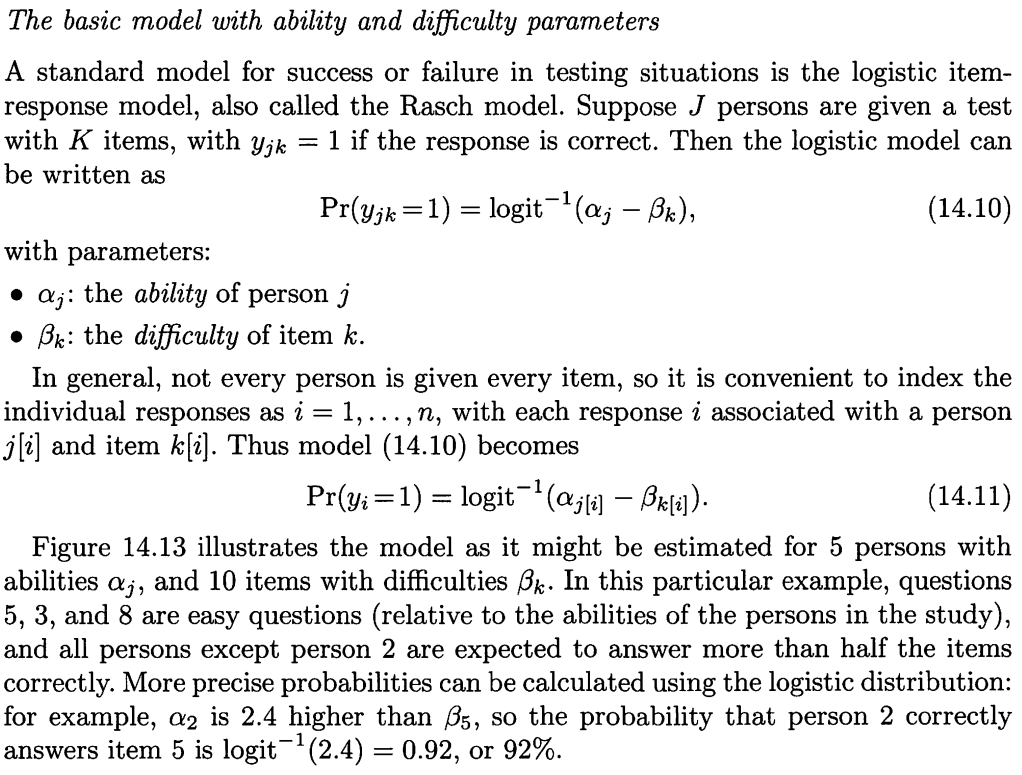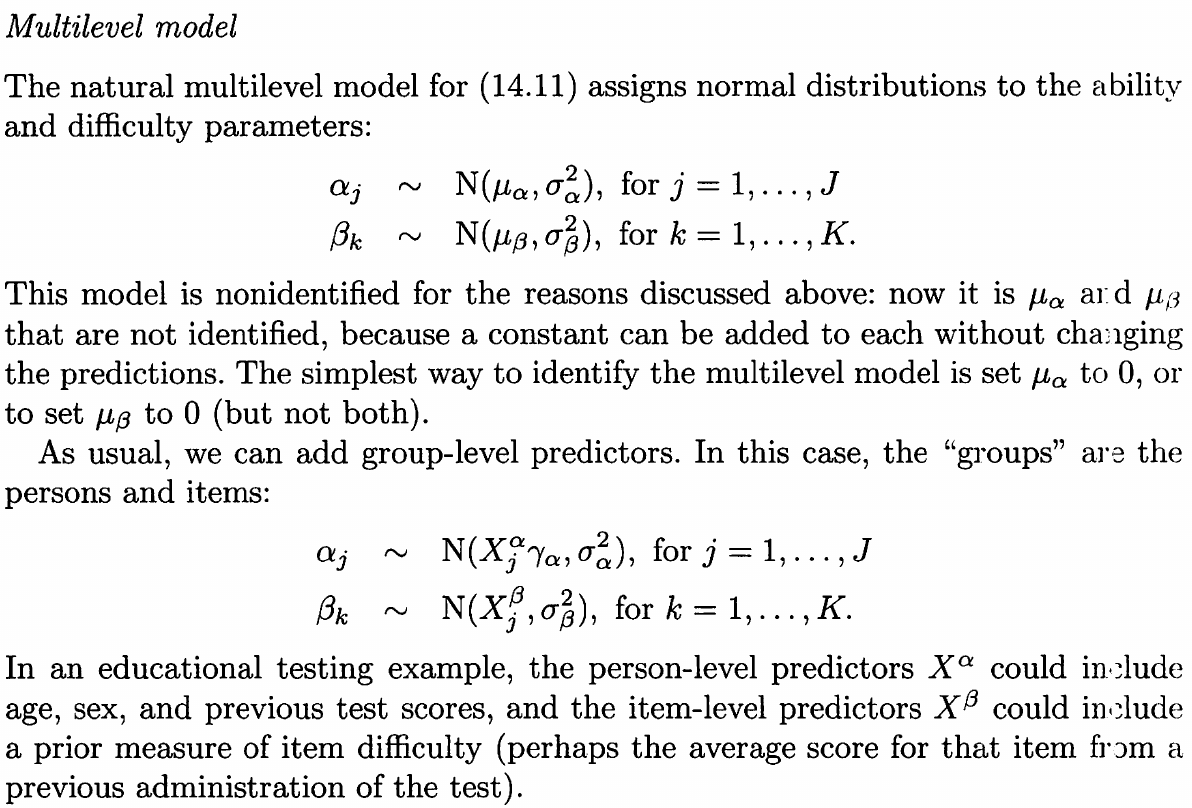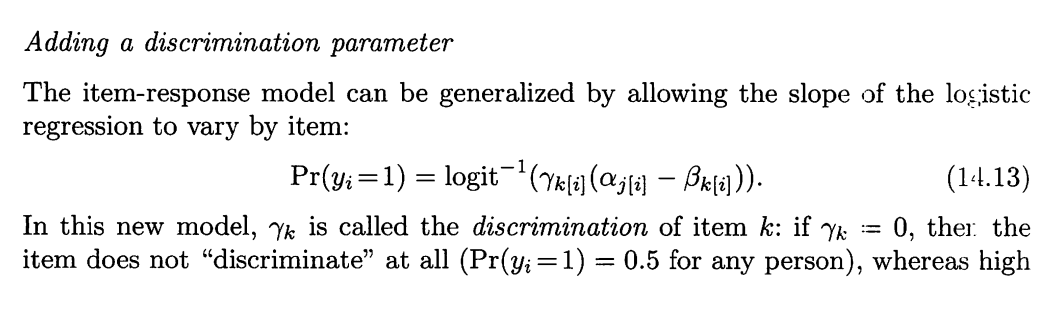### Isotonic regression

The paper points out that the isotonic regression undersmoothes the data and is very sensitive to outlying observations at the endpoints of the design space.

A natural idea is to combine smoothing with isotonic regression, and Mammen (1991) investigates the asymptotic performance of two different strategies,

• $m_{SI}$: first smoothing then isotonic
• $m_{IS}$: first isotonic then smoothing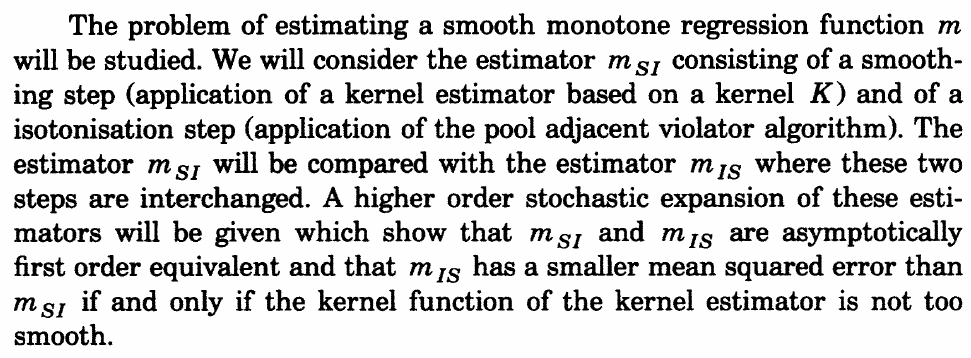### $I$ splines

$I$ splines are obtained by integrating $B$ splines with positive coefficients to ensure monotonicity.

But the class of $I$ splines is relatively small compared to the class of monotone splines, and that there is always a possibilities that the fit to the data could be improved by allowing more general monotone splines.

### Proposal

propose a method based on constrained least absolute deviation principle in the space of B-spline functions

• LAD: can be solved by linear programs
• $L_1$ loss function: the resulting fit approximates the conditional median function rather than the conditional mean

## Method

Suppose $n$ pairs of observations ${(x_i, y_i),i=1,\ldots,n}$.

\begin{equation} y_i = g(x_i) + u_i, i=1,\ldots,n\label{eq:1} \end{equation}

w.l.o.g, restrict $x\in [0, 1]$.

Let $0 = t_0 < t_1 < \cdots < t_{k_n}=1$ be a partition of $[0, 1]$. Let $N=k_n+p=(k_n-1)+(p+1)$ and $\pi_1(x),\ldots, \pi_N(x)$. Choose quadratic splines $p=2$,

$\pi(x) = (\pi_1(x), \pi_2(x), \ldots, \pi_N(x))$

Estimate $g$ by $\hat g_n(x)=\pi(x)^T\hat\alpha$ by minimizing

$\sum_{i=1}^n\vert y_i-\pi(x_i)^T\hat\alpha\vert$

s.t. monotonicity on $\hat g_n$, or equivalently

$\pi'(t_j)^T\alpha\ge 0, j=1,\ldots,k_n$

Rewrite it as

$\sum_{i=1}^n r_i^+ + r_i^-$

subject to

$r_i^+\ge 0, r_i^-\ge 0, r_i^+ - r_i^- = y_i-\pi(x_i)^T\alpha, i=1,\ldots,n$

and

$\pi'(t_j)^T\alpha\ge 0, j=0,\ldots,k_n$

Propose a set of knots equally spaced in percentile ranks.

View the problem of choosing $k$ as model selection to minimize

$IC(k) = \log\left(\sum_i\vert y_i-\hat g_n(x_i)\vert\right) + 2(k+2)/n$

where the factor $2$ is selected mainly due to their experience.

a possible shortcoming is that the degree of freedom should depends on the number of ties in $\alpha$.

The monotone B-spline smoothing generalizes directly to the problem of estimating monotone quantile functions by replace $\vert \cdot \vert$ with

$\rho_\tau(r) = r(\tau - I(r < 0))$

some applications, satisfy certain boundary conditions, such as $0\le g(0)\le g(1)\le 1$ for a probability curve.

## Asymptotic results

Assume that

• model \eqref{eq:1} holds with iid errors
• the knots $t_j$ are nonstochastic and nearly uniformly spaced

In addition, make the following assumptions

• C1: The distribution of the design points are not too far from uniform in [0, 1]. The design points $x_i\in [0, 1] (i=1,\ldots,n)$ satisfy
$\int_0^{x_i} w(t) dt = \frac in + O\left(1/n^2\right), \; 1\le i\le n\,,$

for some continuous function $w$ such that $\int_0^1 w(t)dt = 1$ and $0 < c_1\le w(t) \le c_2<\infty$ for some constants $c_1$ and $c_2$.

• C2: Error distribution. The distribution of $u_i$ has a uniformly bounded, continuous, and strictly positive density $f$ with a uniformly bounded derivative.
• C3: Smoothness of $g$. The regression function $g$ has a uniformly continuous $m$th ($m\ge 2$) order derivative on $[0, 1]$

Assume that conditions C1, C2, and C3 hold. If $k_n \sim (n/\log n)^{1/(2m+1)}$, then with probability one the $B$-spline estimate $\tilde g_n$ of order $m$ or $m+1$ satisfies

$\sup_{x\in [0, 1]}\vert \tilde g_n(x) - g(x)\vert = O\left((\log n/n)^{m/(2m+1)}\right)\,,$

and

$\sup_{x\in [0, 1]}\vert \tilde g_n'(x) - g'(x)\vert = O\left((\log n/n)^{(m-1)/(2m+1)}\right)\,.$

Considering the use of quadratic splines when C3 holds with $m = 2$, the rate of convergence for the constrained $B$-spline estimate $\hat g_n$ is the same as that of the unconstrained one if $g$ is strictly monotone.

Suppose that $g$ is strictly increasing. Let $\varepsilon_0 = \min{g’(x), x\in [0, 1]} > 0$. If $\tilde g_n’$ converges uniformly to $g’$, then as $n$ becomes sufficiently large, say $n > n(\varepsilon_0)$, we have

$\vert \tilde g_n'(t_j) - g'(t_j)\vert < \varepsilon_0/2\,,$

for all knots, then

$\varepsilon_0/2 \le g(t_j) - \varepsilon_0/2 < \tilde g_n'(t_j) < g(t_j) + \varepsilon_0/2$

for all knots. Because $\tilde g_n’$ is piecewise linear, this implies that $\tilde g_n$ is actually monotone (Maybe we can directly conclude by using the uniform convergence on any $x$ instead of knots). In other words, the constrained and the unconstrained $B$-spline estimates are identical when $n > n(\varepsilon_0)$. Hence, the asymptotic properties of the unconstrained $B$-spline estimate $\tilde g_n$ carry over to $\hat g_n$.

Then we directly obtain the first result in the following theorem.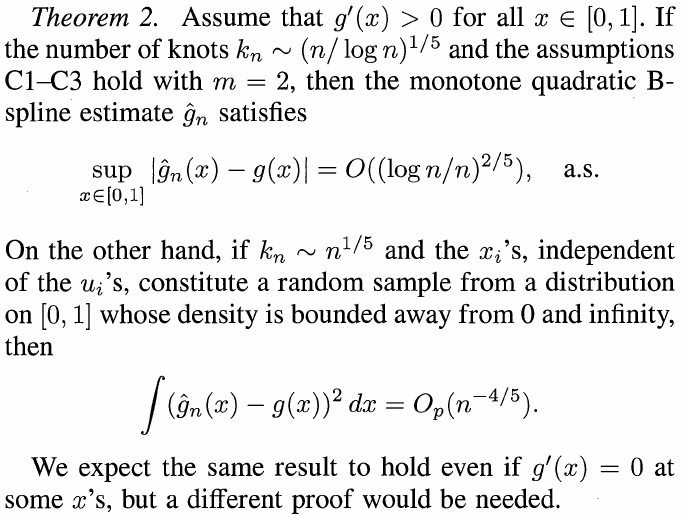The second result comes from the He and Shi (1994),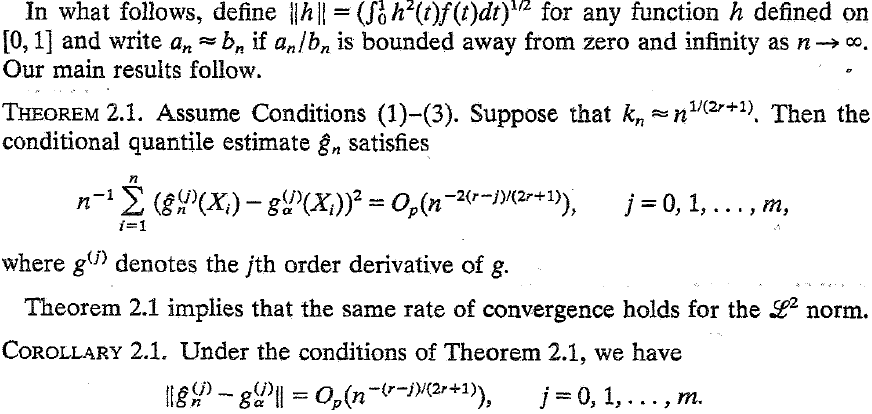where $j = 0, r = 2$.

## Simulations

Compare the root mean squared error (RMSE) with competitors

$\RMSE(q) = \left\{(n-2q)^{-1}\sum_{i=q+1}^{n-q}(\hat g_n(x_i)-g(x_i))^2\right\}^{1/2}$

where $q$ aims to compare the performance in the interior of the design space, and $\RMSE(0)$ is the classical $\RMSE$.

Note that the error term is calculated as $\hat g_n(x_i) - g(x_i)$ instead of $\hat g_n(x_i) - y_i$, where $y_i = g(x_i) + u_i$.

The original competitors are

• Kernel Smoothing with WARPing algorithm, which can automatically determine the bandwidth
• Monotone Kernel, which first perform isotonic regression then smoothing

I did not find available implementation for the WARPing algorithm, and then I pick the Local Polynomial Regression Fitting (loess in R), and also consider $m_{SI}$ in additional to $m_{IS}$.

The implementation of the paper’s method is calling cobs from Ng & Maechler (2007)’s COBS package.

The results are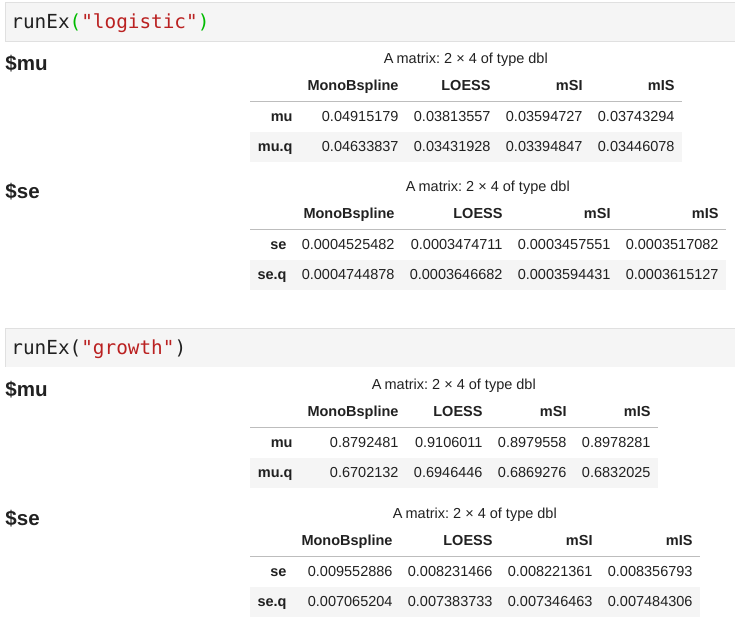Note that the numeric results for the proposed method are quite close to the original paper.

Published in categories Report